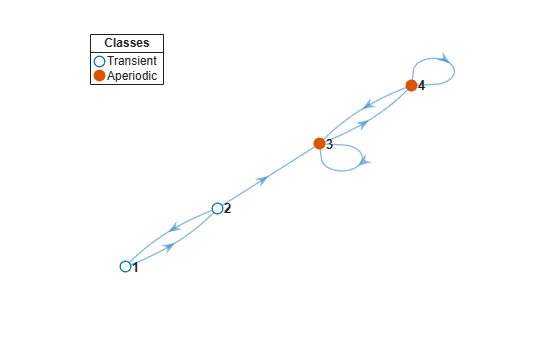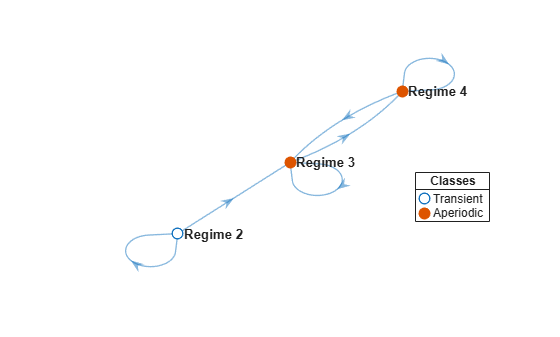# subchain

Extract Markov subchain

## Syntax

``sc = subchain(mc,states)``

## Description

example

``sc = subchain(mc,states)` returns the subchain `sc` extracted from the discrete-time Markov chain `mc`. The subchain contains the states `states` and all states that are reachable from `states`.`

## Examples

collapse all

Consider this theoretical, right-stochastic transition matrix of a stochastic process.

`$P=\left[\begin{array}{cccc}0& 1& 0& 0\\ 0.5& 0& 0.5& 0\\ 0& 0& 0.5& 0.5\\ 0& 0& 0.5& 0.5\end{array}\right].$`

Create the Markov chain that is characterized by the transition matrix P.

```P = [0 1 0 0; 0.5 0 0.5 0; 0 0 0.5 0.5; 0 0 0.5 0.5]; mc = dtmc(P);```

Plot a directed graph of the Markov chain. Visually identify the communicating class to which each state belongs by using node colors.

```figure; graphplot(mc,'ColorNodes',true);```Determine the stationary distribution of the Markov chain.

`x = asymptotics(mc)`
```x = 1×4 0.0000 0.0000 0.5000 0.5000 ```

The Markov chain eventually gets absorbed into states `3` and `4`, and subsequent transitions are stochastic.

Extract the recurrent subchain of the Markov chain by passing `mc` to `subchain` and specifying one of the states in the recurrent, aperiodic communicating class.

`sc = subchain(mc,3);`

`sc` is a `dtmc` object.

Plot a directed graph of the subchain.

```figure; graphplot(sc,'ColorNodes',true)```Consider this theoretical, right-stochastic transition matrix of a stochastic process.

`$P=\left[\begin{array}{cccc}0.5& 0.5& 0& 0\\ 0& 0.5& 0.5& 0\\ 0& 0& 0.5& 0.5\\ 0& 0& 0.5& 0.5\end{array}\right].$`

Create the Markov chain that is characterized by the transition matrix P. Name the states Regime 1 through Regime 4.

```P = [0.5 0.5 0 0; 0 0.5 0.5 0; 0 0 0.5 0.5; 0 0 0.5 0.5]; mc = dtmc(P,'StateNames',["Regime 1" "Regime 2" "Regime 3" "Regime 4"]);```

Plot a digraph of the chain. Visually identify the communicating class to which each state belongs by using node colors.

```figure; graphplot(mc,'ColorNodes',true);```Regimes 1 and 2 are in their own communicating class because Regime 2 does not transition to Regime 1.

Extract the subchain containing Regime 2, a transient state. Display the transition matrix of the subchain.

```sc = subchain(mc,"Regime 2"); sc.P```
```ans = 3×3 0.5000 0.5000 0 0 0.5000 0.5000 0 0.5000 0.5000 ```

Regime 1 is not in the subchain.

Plot a digraph of the subchain.

```figure; graphplot(sc,'ColorNodes',true);```The plot shows a unichain: a Markov chain containing one recurrent communicating class and the selected transient class.

## Input Arguments

collapse all

Discrete-time Markov chain with `NumStates` states and transition matrix `P`, specified as a `dtmc` object. `P` must be fully specified (no `NaN` entries).

States to include in the subchain, specified as a numeric vector of positive integers, string vector, or cell vector of character vectors.

• For a numeric vector, elements of `states` correspond to rows of the transition matrix `mc.P`.

• For a string vector or cell vector of character vectors, elements of `states` must be state names in `mc.StateNames`.

Example: `["Regime 1" "Regime 2"]`

Data Types: `double` | `string` | `cell`

## Output Arguments

collapse all

Discrete-time Markov chain, returned as a `dtmc` object. `sc` is a subchain of `mc` containing the states `states` and all states reachable from `states`. The state names of the subchain `sc.StateNames` are inherited from `mc`.

## Algorithms

• State `j` is reachable from state `i` if there is a nonzero probability of moving from `i` to `j` in a finite number of steps. `subchain` determines reachability by forming the transitive closure of the associated digraph, then enumerating one-step transitions.

• Subchains are closed under reachability to ensure that the transition matrix of `sc` remains stochastic (that is, rows sum to `1`), with transition probabilities identical to the transition probabilities in `mc.P`.

• If you specify a state in a recurrent communicating class, then `subchain` extracts the entire communicating class. If you specify a state in a transient communicating class, then `subchain` extracts the transient class and all classes reachable from the transient class. To extract a unichain, specify a state in each component transient class. See `classify`.

 Gallager, R.G. Stochastic Processes: Theory for Applications. Cambridge, UK: Cambridge University Press, 2013.

 Horn, R., and C. R. Johnson. Matrix Analysis. Cambridge, UK: Cambridge University Press, 1985.